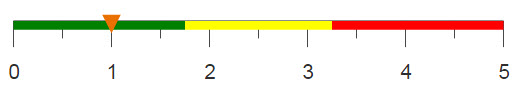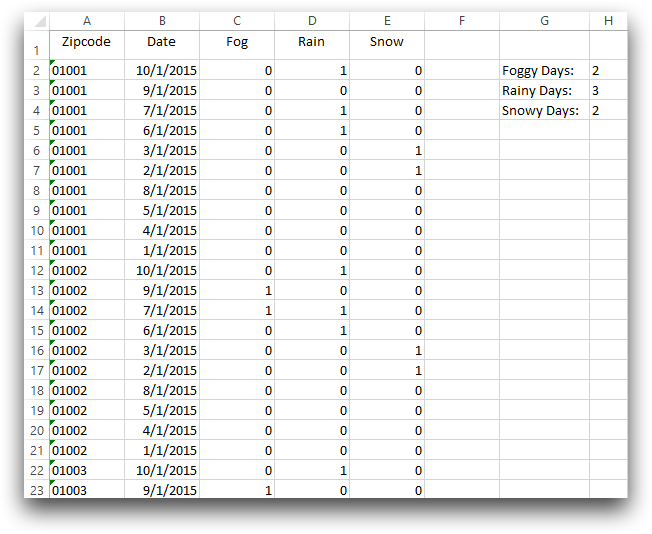# Summing over multiple conditions

G_functions allow you to calculate multiple sums based on data meeting certain criteria, similar to SUMIFS in Excel.

## Difficulty## Objective

You would like to sum several different sets of values based on various criteria. However, these sets are contained all in one table and the different values are distributed throughout the table instead of being organized by groups. In Excel you can use the SUMIFS function to set conditions for which data you would life to sum. With 1010data, you can solve this issue by using the if function in tandem with the g_sum function, or you can use the g_sum function along with a flag column to denote the values you would like to include in your summation.

## Excel solution

The SUMIFS function in Excel, sums the values in a column based on corresponding columns containing specified values. In this recipe, the Observed Daily Weather data is used to sum the total number of rainy, foggy and snowy days based on a specified zip code. For example, if you wanted to calculate these sums for the zip code 01002, you would enter the following formulas in an Excel worksheet.

`=SUMIFS(\$C\$2:\$C\$101,\$A\$2:\$A\$101,1002,\$C\$2:\$C\$101,1)`
`=SUMIFS(\$D\$2:\$D\$101,\$A\$2:\$A\$101,1002,\$D\$2:\$D\$101,1)`
`=SUMIFS(\$E\$1:\$E\$100,\$A\$1:\$A\$100,1002,\$E\$1:\$E\$100,1)`

The first condition corresponds to the column, or the data, you want summed, while the following conditions depict where to look for the criteria each value must meet and what the criteria is, respectively. With this function, you can have an unlimited number of criteria conditions. The image below shows the data in Excel along with the cells containing the calculated sums.For each sum you want to compute, if it is based on a different set of criteria, you need to repeat this formula in another cell with the conditions changed. However, with 1010data's platform you can perform several sums each based on different criteria, in the same equation. Additionally, Excel is limited to the amount of data it can process, while 1010data can process larger amounts of data than Excel and at a faster speed.

## 1010data Macro Language solution

```<base table="pub.demo.weather.wunderground.observed_daily"/>
<sel value="year(date)=2015 & day(date)=01"/>
<sel value="i_<101"/>
<colord cols="zipcode,date,fog,rain,snow,sum"/>
<willbe name="sum" value="if(zipcode=01002&rain=1;
splice('rain',g_sum(zipcode;;rain);'-');zipcode=01002&fog=1;
splice('fog',g_sum(zipcode;;fog);'-');zipcode=01002&snow=1;
splice('snow',g_sum(zipcode;;snow);'-');NA)"/>```

The if(C1;R1;C2;R2;...;D) function allows for multiple conditions and also provides an option in the case that none of these conditions are met. The first condition relates what information you want to match, and the second condition describes what to do if this criteria is met.

For this analogue, the transaction sum is calculated if the store is equal to 15 and if the department is 36. You calculate the sum using g_sum(G;S;X), where G is the list of columns to denote which values are in the same group, and X is the column that you want summed.

Although the if function is not the most efficient function, it allows us to calculate multiple sums with one continuous line of code and have all of the results appear in one column.

## Alternate 1010data Macro Language solution

```<base table="pub.demo.weather.wunderground.observed_daily"/>
<sel value="year(date)=2015 & day(date)=01"/>
<sel value="i_<101"/>
<colord cols="zipcode,date,fog,rain,snow,sum"/>
<willbe name="flagr" value="zipcode=01002&rain=1"/>
<willbe name="flagf" value="zipcode=01002&fog=1"/>
<willbe name="flags" value="zipcode=01002&snow=1"/>
<willbe name="rsum" label="Sum of Rainy Days"
value="g_sum(zipcode;flagr;rain)"/>
<willbe name="fsum" label="Sum of Foggy Days"
value="g_sum(zipcode;flagf;fog)"/>
<willbe name="ssum" label="Sum of Snowy Days"
value="g_sum(zipcode;flags;snow)"/>```

Alternatively, you can utilize the S parameter in the g_sum function which denotes a flag, or selection column. This column will contain either a 1 or a 0 to denote whether or not that row of data should be included in the final calculation. You create this reference column(s) using a willbe operation where the value is equal to the criteria. This solution is more efficient but creates six columns instead of just one, as in the previous solution.

## Further reading

If you would like to learn more about the functions and operations discussed in this recipe, click on the links below:

if(C1;R1;C2;R2;...;D)

g_sum(G;S;X)

splice(X;Y)